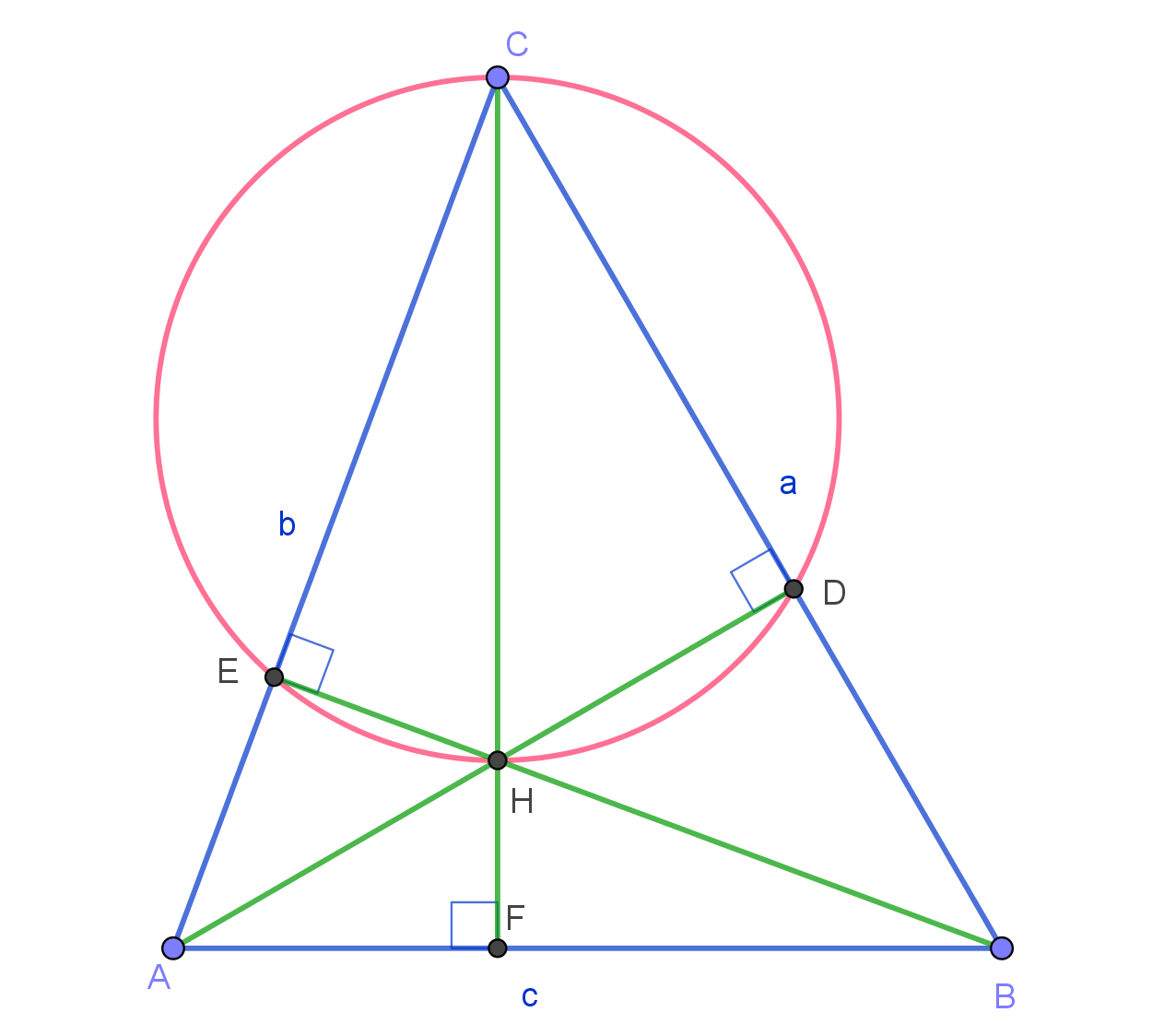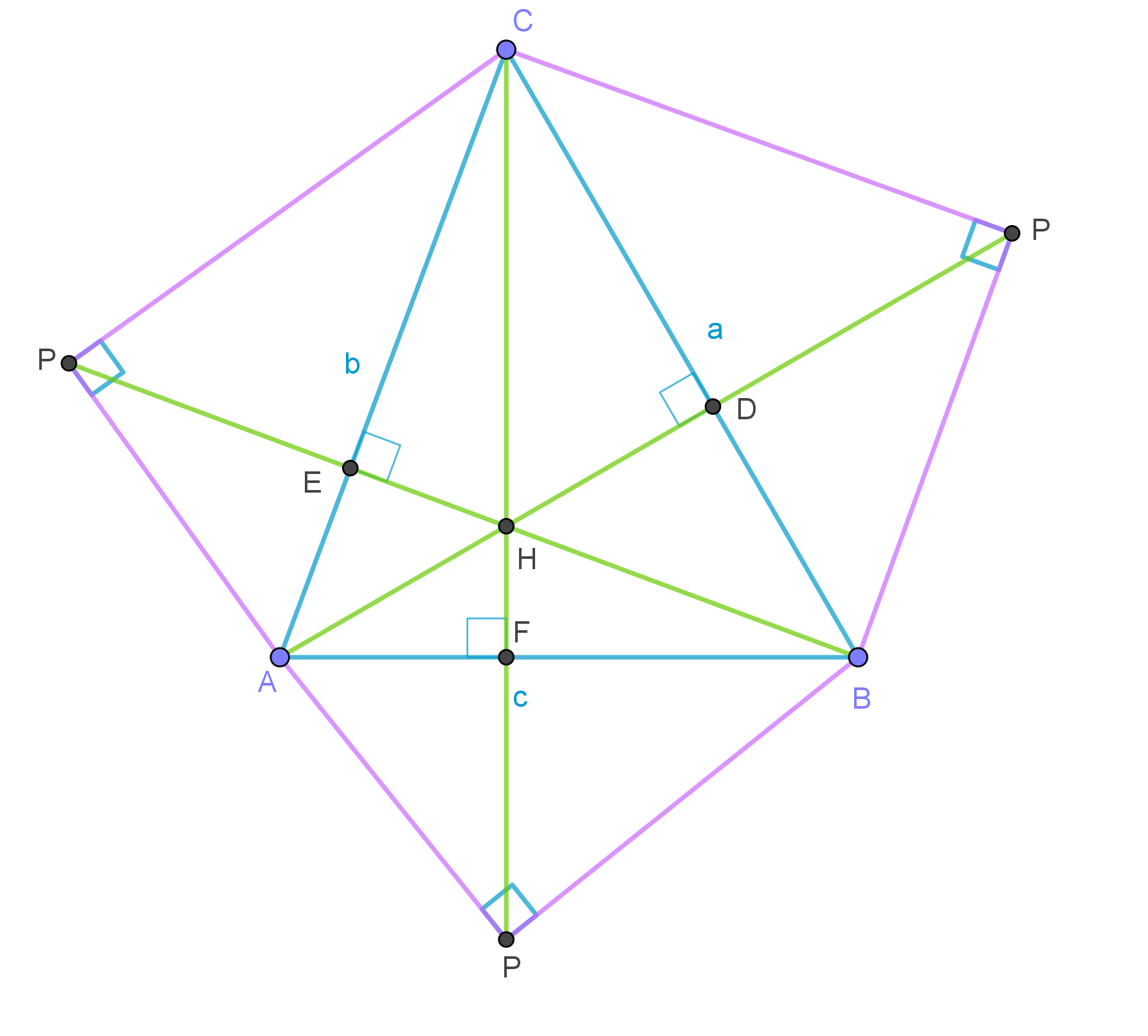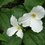# Generalization of Pythagorean Theorem with Orthocentre

We all know Pythagorean Theorem: the legs a, b and a hypotenuse $c$ of any right triangle satisfy an equation: $a^2 + b^2 = c^2$

The commonly known generalization of that theorem is Law of Cosines:

$c^2=a^2 + b^2 - 2 a \times b \times cos(C)$

There is another generalization and it involves use of Orthocentre. Let $H$ be an Orthocentre of $\triangle ABC$, let $D,E,F$ denote feet of altitudes from $A,B,C$ respectively.$cos(C) = \dfrac{CE}{a} = \dfrac{CD}{b}$

When we use that as a substitution in the Law of Cosines we obtain: $c^2 = a(a-CD) + b(b-CE) = a \times BD + b \times AE$ or $AB^2 = AC \times AE+ BC \times BD$

Since $\angle ADE = 90, \angle BEC = 90$ we conclude that quadrilateral $CEHD$ is cyclic. Using power of point we can state that: $AE \times AC = AH \times AD$ and $BD \times BC = BH \times BE$

Susbstituting it in the previous equation we get

$AB^2 = AH \times AD+ BH \times BE$

In a special case when $\angle C = 90$ then $H=C=D=E$ and it becomes regular Pythagorean Theorem.

What is interesting is that if we create a right triangle on the other side of $AB$, with legs $AP=\sqrt{ AH \times AD}, BP= \sqrt{ BH \times BE}$ and hypotenuse $AB$, and repeat for sides $BC, AC$ we will get a net of a orthogonal tetrahedron with apex $P$.Orthogonal projection of apex $P$ onto $\triangle ABC$ is orthocentre $H$. Projection of $AP$ is $AH$ etc.

It is commonly known that $AH \times HD= BH \times HE = CH \times HF$.

It can be easily shown when exploring tetrahedron $ABCP$ that this constant is $HP^2$.

$HP^2 = AH \times HD= BH \times HE = CH \times HF$

PS

I currently do not have time to fill out all the details but I trust anybody who knows basics of geometry can fill in the blanks. I have come into realization of these facts which amuse me some years ago when solving brilliant Trirectangular Corner Locus and eventually I made an effort to write this as imperfect as it is. I thought it is better than not doing it at all. Writing, even notes or solutions can always be perfected, but fear of imperfection often means not doing it at all.Note by Maria Kozlowska
2 years, 8 months ago

This discussion board is a place to discuss our Daily Challenges and the math and science related to those challenges. Explanations are more than just a solution — they should explain the steps and thinking strategies that you used to obtain the solution. Comments should further the discussion of math and science.

When posting on Brilliant:

• Use the emojis to react to an explanation, whether you're congratulating a job well done , or just really confused .
• Ask specific questions about the challenge or the steps in somebody's explanation. Well-posed questions can add a lot to the discussion, but posting "I don't understand!" doesn't help anyone.
• Try to contribute something new to the discussion, whether it is an extension, generalization or other idea related to the challenge.

MarkdownAppears as
*italics* or _italics_ italics
**bold** or __bold__ bold
- bulleted- list
• bulleted
• list
1. numbered2. list
1. numbered
2. list
Note: you must add a full line of space before and after lists for them to show up correctly
paragraph 1paragraph 2

paragraph 1

paragraph 2

[example link](https://brilliant.org)example link
> This is a quote
This is a quote
    # I indented these lines
# 4 spaces, and now they show
# up as a code block.

print "hello world"
# I indented these lines
# 4 spaces, and now they show
# up as a code block.

print "hello world"
MathAppears as
Remember to wrap math in $$ ... $$ or $ ... $ to ensure proper formatting.
2 \times 3 $2 \times 3$
2^{34} $2^{34}$
a_{i-1} $a_{i-1}$
\frac{2}{3} $\frac{2}{3}$
\sqrt{2} $\sqrt{2}$
\sum_{i=1}^3 $\sum_{i=1}^3$
\sin \theta $\sin \theta$
\boxed{123} $\boxed{123}$

Sort by:

May I ask whether you have written any papers on it? I am writing a paper on orthocentric tetrahedra and would really like to look into this aspect of it.

- 2 years, 8 months ago

No, not yet,

- 2 years, 8 months ago

Some notes:

1. At the point of substitution of the results of $\cos(C)$ into the Law of Cosines, you will need to expand on how you arrived at the answer. You cannot expect people to get exactly what you are talking about, especially when you jumped at least 3-4 steps.

2. I think what you are trying to get at is the Power of a point theorem; you will need to explain clearly what this is. Again, you can't just expect people to know what you are talking about if you're not willing to define your terms properly; a link would generally suffice.

3. Nice observation with regards to the net of an orthocentric tetrahedron! We can see this to be true because the sum of squares of opposite edges of such orthocentric tetrahedron would be equal, by the eighth equation (you may want to number your equations for cleanliness, but I normally don't do this).

However, I don't see how this is a generalisation of Pythagoras' theorem using orthocentres. All you have done so far is use the orthocentre of a triangle to generate a net for an orthocentric tetrahedron...

P.S. If it takes me more than 10 minutes to try to understand this note, then I generally consider there to be something wrong with it. In general, if you lay out your steps properly, concisely and in detail, you will find that it would save the reader a heap of time to understand what you are writing.

- 2 years, 8 months ago